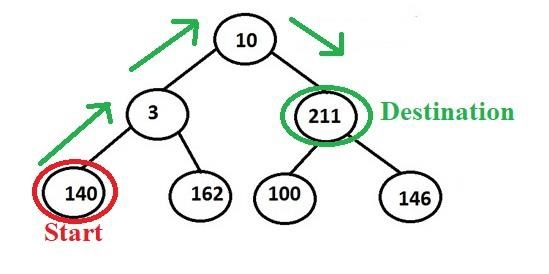# Print path between any two nodes in a Binary Tree in C++ Programming.

We are given with a binary tree of distinct nodes and two nodes of the binary tree whose path in the binary tree we want to print.For Example − We want to print the path between node 140 to 211 so its output should be like −

Output: 140->3->10->211

The idea is to find paths form root nodes to the two nodes and store them in two separate vectors or arrays say path1 and path2.

Now, there arise two different cases −

• If the two nodes are in different subtrees of root nodes − When the two nodes are in different subtrees like one in left and other in right. In this case, it is clear that the root node will lie in between the path from node1 to node2. So, print path1 in reverse order and then path2.
• If the nodes are in the same subtree − When both of the nodes are in the same subtree that can be either in the left subtree or in the right subtree. In this case, you need to observe the path from the root to the two nodes will have an intersection point before which the path is common for both the two nodes from the root node. We have to find the intersection point and print nodes from that point like the above case.

## Algorithm

START
STEP 1-> DEFINE A struct Node
WITH int data AND Node *left, *right;
STEP 2-> DEFINE A TREE STRUCTURE struct Node* tree(int data)FUNCTION bool path(Node* root, vector& arr, int x)
STEP 1-> IF root IS NULL
RETURN false
END IF
STEP 2-> arr.push_back(root->data)
IF root->data == x THEN
RETURN true
END IF
STEP 3-> IF path(root->left, arr, x) || path(root->right, arr, x) THEN,
RETURN true
STEP 4-> arr.pop_back()
return false
END FUNCTION
FUNCTION void printPath(Node* root, int n1, int n2)
STEP 1-> DEFINE A vector<int> path1
STEP 2-> DEFINE A vector<int> path2
STEP 3-> CALL FUNCTION path(root, path1, n1)
STEP 4-> CALL FUNCTION path(root, path2, n2)
STEP 5-> DEFINE AND SET intersection = -1, i = 0, j = 0
STEP 6-> LOOP WHILE i != path1.size() || j != path2.size()
IF i == j && path1[i] == path2[j]
INCREMENT i BY 1
INCREMENT j BY 1
ELSE
SET intersection = j - 1
BREAK;
END IF
END WHILE
STEP 7-> LOOP FOR i = path1.size() – 1 AND i > intersection AND i--PRINT path1[i]
END FOR
STEP 8-> LOOP FOR i = intersection AND i < path2.size() AND i++
PRINT path2[i]
END FOR

## Example

Live Demo

#include <bits/stdc++.h>
using namespace std;
// structure of a node of binary tree
struct Node {
int data;
Node *left, *right;
};
struct Node* tree(int data){
struct Node* newNode = new Node;
newNode->data = data;
newNode->left = newNode->right = NULL;
return newNode;
}
bool path(Node* root, vector<int>& arr, int x){
if (!root)
return false;
// push the node's value in 'arr'
arr.push_back(root->data);
// if it is the required node
// return true
if (root->data == x)
return true;
if (path(root->left, arr, x) || path(root->right, arr, x))
return true;
arr.pop_back();
return false;
}
// Function to print the path between
// any two nodes in a binary tree
void printPath(Node* root, int n1, int n2){
// vector to store the path
vector<int> path1;
vector<int> path2;
path(root, path1, n1);
path(root, path2, n2);
int intersection = -1;
int i = 0, j = 0;
while (i != path1.size() || j != path2.size()) {
if (i == j && path1[i] == path2[j]) {
i++;
j++;
} else {
intersection = j - 1;
break;
}
}
// Print the required path
for (int i = path1.size() - 1; i > intersection; i--)
cout << path1[i] << " ";
for (int i = intersection; i < path2.size(); i++)
cout << path2[i] << " ";
}
int main(){
// binary tree formation
struct Node* root = tree(1);
root->left = tree(2);
root->left->left = tree(4);
root->left->left->left = tree(6);
root->left->right = tree(5);
root->left->right->left = tree(7);
root->left->right->right = tree(8);
root->right = tree(3);
root->right->left = tree(9);
root->right->right = tree(10);
int node1 = 5;
int node2 = 9;
printPath(root, node1, node2);
return 0;
}

## Output

This Program will Print output −

5 2 1 3 9